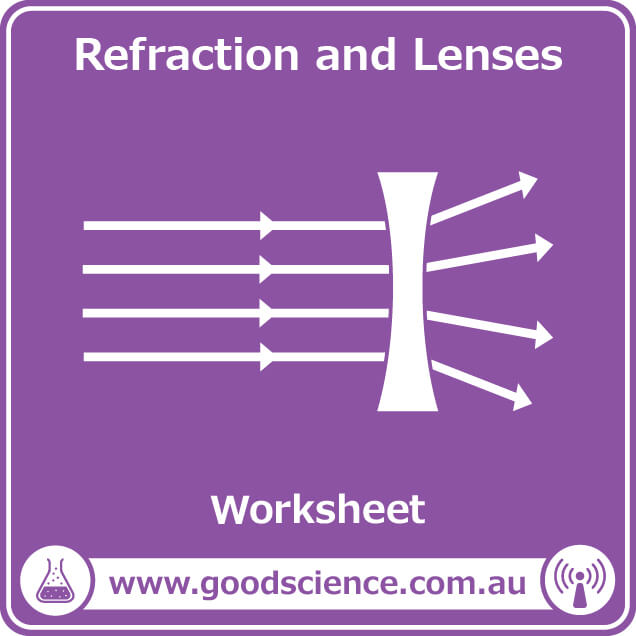# Refraction and Lenses – Worksheet

\$12.00

Worksheet to accompany the lesson Refraction and Lenses.

Click cover for full preview. Scroll down for product information.Categories: , ,

## Description

Worksheet Contents

• This worksheet covers:
• Refraction
• The refractive index
• The law of refraction (Snell’s law)
• Convex and concave lenses
• Refraction of light by convex and concave lenses
• Images formed by convex and concave lenses
• Lens curvature and focal length
• Lenses in the eye
• Properties of symmetrical lenses
• Drawing ray diagrams
• Real images and virtual images
• Includes:
• Unit outline.
• Cloze activity (fill in the blanks).
• Extension questions.
• Topics for further research.
• Teacher version containing complete answers.
• Aligned to the Australian Year 9 Science curriculum.
• 12 pages.

Learning Outcomes

• By the end of this worksheet students will be able to:
• ☑   Explain refraction and describe the refractive index, using water and prisms as examples.
• ☑   Describe the law of refraction.
• ☑   Describe convex and concave lenses.
• ☑   Describe how light refracts through convex and concave lenses.
• ☑   Define focal point, focal length, principal axis, mid-plane and optical centre.
• ☑   Describe how lenses in the eye focus light.
• ☑   Differentiate between near-sightedness and far-sightedness and describe how they can be corrected with lenses.
• ☑   Draw ray diagrams to determine the images produced by convex and concave lenses.
• ☑   Differentiate between real and virtual images produced by lenses.

This resource is also included in the Year 9 Physics PDF Workbook.